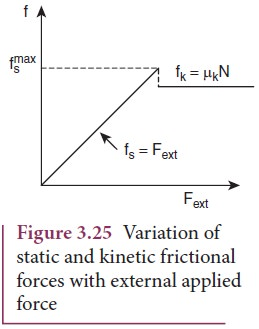Home | | Physics 11th std | Kinetic Friction

# Kinetic Friction

If the external force acting on the object is greater than maximum static friction, the objects begin to slide.

Kinetic Friction

If the external force acting on the object is greater than maximum static friction, the objects begin to slide. When an object slides, the surface exerts a frictional force called kinetic friction(also called sliding friction or dynamic friction). To move an object at constant velocity we must apply a force which is equal in magnitude and opposite to the direction of kinetic friction.Experimentally it was found that the magnitude of kinetic friction satisfies the relationwhere µk is the coefficient of kinetic friction and N the normal force exerted by the surface on the object,This implies that starting of a motion is more difficult than maintaining it. The salient features of static and kinetic friction are given in Table 3.2.The variation of both static and kinetic frictional forces with external applied force is graphically shown in Figure 3.25.The Figure 3.25 shows that static friction increases linearly with external applied force till it reaches the maximum. If the object begins to move then the kinetic friction is slightly lesser than the maximum static friction. Note that the kinetic friction is constant and it is independent of applied force.

Study Material, Lecturing Notes, Assignment, Reference, Wiki description explanation, brief detail
11th Physics : UNIT 3 : Laws of Motion : Kinetic Friction |